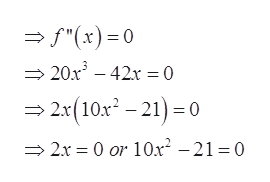Use a graphing calculator to estimate the x-coordinates of the inflection points of the function, rounding your answers to two decimal places. [Hint: Graph the second derivative, either calculating it directly or using NDERIV twice, and see where it crosses the x-axis. Enter your answers as a comma-separated list.]f(x) = x5 − 7x3 + 6x + 2

Question

Use a graphing calculator to estimate the x-coordinates of the inflection points of the function, rounding your answers to two decimal places. [Hint: Graph the second derivative, either calculating it directly or using NDERIV twice, and see where it crosses the x-axis. Enter your answers as a comma-separated list.]
f(x) = x5 − 7x3 + 6x + 2

Step 1

Given,

Step 2

By calculation, derivative of f(x) are

Step 3

For inflection points, second derivat...help_outlineImage Transcriptionclose"(x)0 20x3 42=0 2x(10x-21)0 0 or 10x2 - 21 = 0 2x fullscreen

Want to see the full answer?

See Solution

Want to see this answer and more?

Our solutions are written by experts, many with advanced degrees, and available 24/7

See Solution
Tagged in

Functions